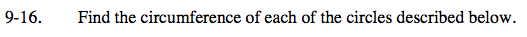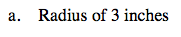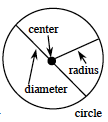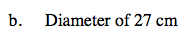Home > ACC7 > Chapter cc29 > Lesson cc29.1.1 > Problem9-16

9-16.
1. Find the circumference of each of the circles described below. Homework Help ✎

1. Radius of 3 inches

2. Diameter of 27 cmRecall that the circumference of a circle = 2π(radius).2π(3 inches) = 6π ≈ 18.84 inchesRefer to the diagram from part (a) and recall that the circumference = π(diameter).

27π
To get an appropriate decimal answer, round π so that it equals 3.14